## Comparing Unlike Fractions and Least Common Multiple Part 1

Practice Unlimited Questions

#### LESSON AT A GLANCE

This lesson covers the following:

#### 1. What are Like Fractions?

Like Fractions are two or more fractions that have the same denominator.

Example:
 1 5
and
 2 5
are like fractions. Both these fractions have the same denominator of 5.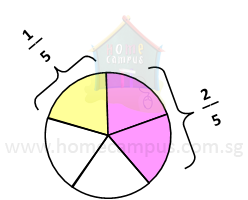#### 2. What are Unlike Fractions?

Unlike Fractions are two or more fractions that do not have the same denominator.

Example:
 1 3
and
 1 4
are unlike fractions. The denominators of these two fractions are different.

#### 3. Which of the following fractions is greater?

 1 3
or
 1 4
 1 3
and
 1 4
can be represented pictorially as below, on two different fraction strips.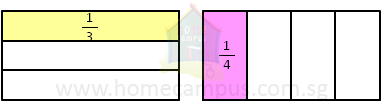In order to compare the two fractions, we must be able to represent them on a single fraction strip. This means we should first change them to like fractions using the following steps:

Step 1:
Find the product of 3 and 4 (the two denominators).
3  ×  4  =  12

Step 2:
Change each of the two fractions to its equivalent fraction so that the new denominator is 12.
 1 3
=
 ? 12
=>
 1 x 4 3 x 4
=
 4 12

 1 4
=
 ? 12
=>
 1 x 3 4 x 3
=
 3 12

Now that both the fractions have the same denominator, we can show them on one fraction strip as below.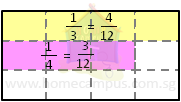Comparing the two fractions
From the above figure, it is clear that,
 4 12
is greater than
 3 12

Or,

 1 3
is greater than
 1 4

#### 4. Which of the following fractions is greater? 1 6   or      1 4

Step 1:
Find the product of 6 and 4 (the two denominators).
6  ×  4  =  24

Step 2:
Change each of the two unlike fractions to its equivalent so that the new denominator is 24.
 1 6
=
 ? 24
=>
 1 x 4 6 x 4
=
 4 24

 1 4
=
 ? 24
=>
 1 x 6 4 x 6
=
 6 24

The two fractions can be shown pictorially as below: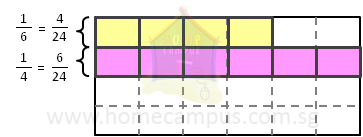Comparing the two fractions
From the above figure, it is clear that,
 6 24
is greater than
 4 24

Or,

 1 4
is greater than
 1 6

#### 5. What is LCM or Least Common Multiple? 1 6   or      1 4

While the above method of converting unlike fractions to like fractions works perfectly with small numbers, there is another method we use when dealing with more than two numbers or with bigger numbers. It is known as LCM or least common multiple.
Example: To find the LCM of 6 and 4, we follow the steps below.

Step 1:
Find the multiples of 6 and the multiples of 4.
6  =>  6, 12, 18, 24, 30, 36, 42, 48, 54, 60, 66, ...
4  =>  4, 8, 12, 16, 20, 24, 28, 32, 36, 40, 44, ...

Step 2:
Find the common multiples of 6 and 4.
They are: 12, 24, 36, ...

Step 3:
Find the least (or smallest) of the common multiples of 6 and 4.
It is 12.
So, the LCM of 6 and 4 is 12.
To find out the multiples of a number, think of the multiplication table of that number.

The multiplication table of 6 is:
6  ×  1 = 6
6  ×  2 = 12
6  ×  3 = 18
6  ×  4 = 24
6  ×  5 = 30
...

Therefore, the multiples of 6 are:
6, 12, 18, 24, 30, ...

#### 6. Using LCM, find which of the following fractions is greater: 1 6   or      1 4

LCM or least common multiple, as we mentioned above, is a way to find out a new common denominator for the given unlike fractions so they can be changed to like fractions.

Step 1:
Take the two unlike denominators, 6 and 4, and find a new common denominator using LCM.

Step 2:
We know from above that the LCM of 6 and 4 = 12.
Step 3:
Change the two unlike fractions so each has a denominator of 12.

 1 6
=
 ? 12
=>
 1 x 2 6 x 2
=
 2 12

 1 4
=
 ? 12
=>
 1 x 3 4 x 3
=
 3 12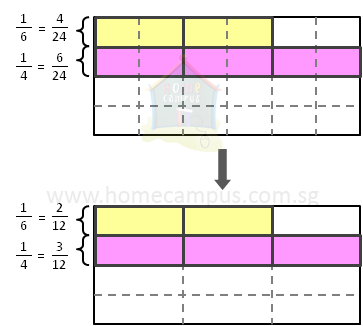This tells us we need not cut the fraction strip into 24 pieces (6  ×  4).

It is sufficient to cut it up into 12 pieces and still be able to mark the unlike fractions
 1 6
and
 1 4
on it for comparison.